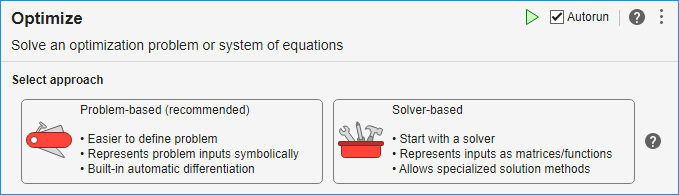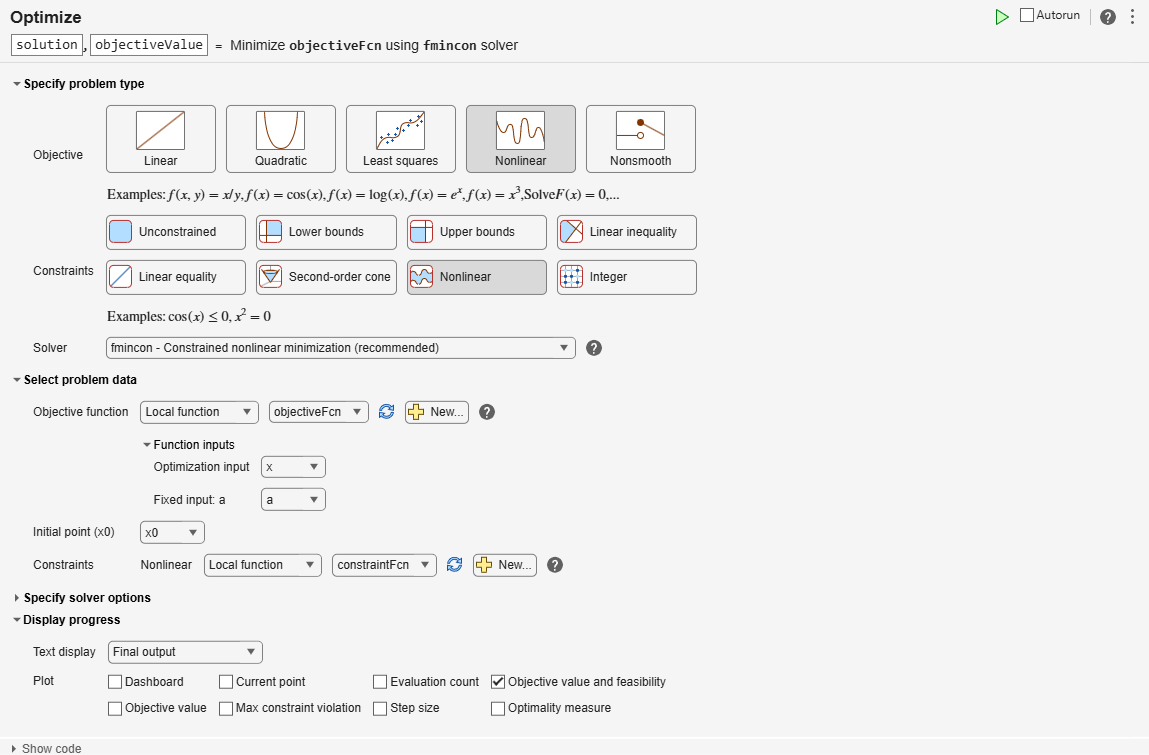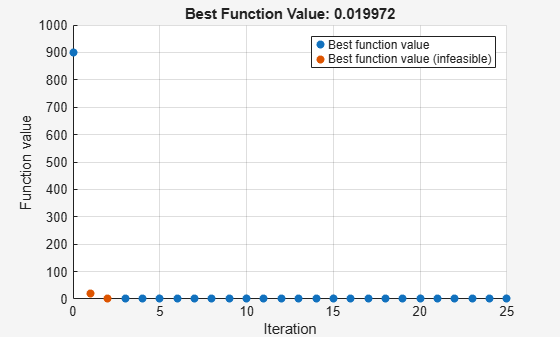# Get Started with Solver-Based Optimize Live Editor Task

This example script helps you to use the solver-based Optimize Live Editor task for optimization or equation solving. Modify the script for your own problem.

The script solves a nonlinear optimization problem with nonlinear constraints.

### Include Parameters or Data

Typically, you have data or values to pass to the solver. Place those values in the input section (where you see `x0`) and run the section by choosing Section > Run Section or pressing `Control+Enter`.

Set the initial point `x0` and scale `a` for the optimization.

```x0 = [2;1]; a = 100;```

Place the `x0` value and any other problem data into the workspace by running this section before proceeding.

Usually, you place the Optimize Live Editor task into the script by selecting Task > Optimize in the Live Editor tab, or by selecting Task > Optimize in the Insert tab. Then you are presented with the following choice (this is only a picture, not the real task):To get the solver-based task, click Solver-based.

The following solver-based task has objective and nonlinear constraint functions included. To change these functions, edit the function listings below the task.

To change the constraints, select appropriate constraint types and enter values in the input boxes. You might need to enter values in the section containing `x0` above, and run the section to put values in the workspace.

Run the task by clicking the striped bar to the left, or by choosing Run or Section > Run Section, or by pressing `Control+Enter`.

`````````Local minimum found that satisfies the constraints. Optimization completed because the objective function is non-decreasing in feasible directions, to within the value of the optimality tolerance, and constraints are satisfied to within the value of the constraint tolerance. ```

### Results

`Optimize` saves the solution to the workspace variable `solution`, and saves the objective function value at the solution to the workspace variable `objectiveValue`. You can see and modify these variable names at the top of the `Optimize` task.

View these variables.

`solution`
```solution = 2×1 1.1413 1.3029 ```
`objectiveValue`
```objectiveValue = 0.0200 ```

View the nonlinear constraint function values at the solution.

`[ccons,ceqcons] = constraintFcn(solution)`
```ccons = 1×2 -2.0000 -0.0000 ```
```ceqcons = [] ```

### Helper Functions — Local Functions

The following code creates the objective function. Modify this code for your problem.

```function f = objectiveFcn(x,a) f = a*(x(2) - x(1)^2)^2 + (1 - x(1))^2; end```

The following code creates the constraint function. Modify this code for your problem.

```function [c,ceq] = constraintFcn(x) c(1) = x(1)^2 + x(2)^2 - 5; c(2) = 3 - x(1)^2 - x(2)^2; ceq = []; % No equality constraints end```